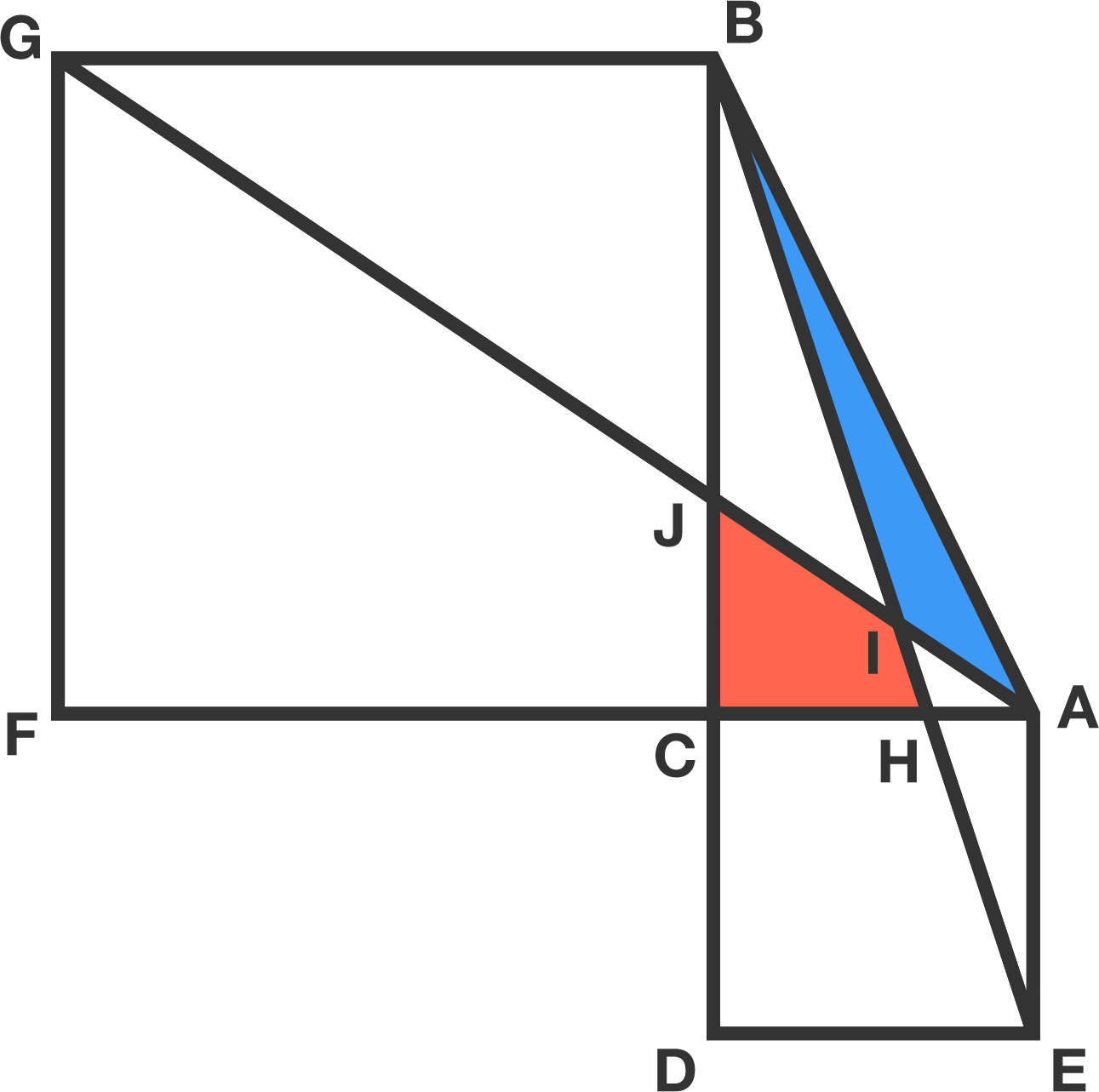# Problems of the Week

Contribute a problem

# 2018-03-19 Intermediate

In a certain country, each couple stops having children if and only if they have a female. After many generations, what will the sex ratio in this country be?Details and Assumptions:

• The only exception to the rule stated above is for multiple births (twins, triplets, etc.). After a multiple birth with any number of female children, the couple stops having children.
• Assume that all couples are capable of having female children, and that the chance of either gender is equal for each birth.
• Assume that life expectancy is the same for males and females.

$21^{100}-12^{100}$

Is this result divisible by $11?$

$ACDE$ and $BGFC$ are both squares and $\angle BCA = 90^{\circ}.$

How do the blue and red areas compare?An Egyptian mummy is carbon-dated and compared with a scroll found in the Dead Sea, which is known to be 2000 years old. The $\ce{^{14}C} \text{ : } \ce{^{12}C}$ ratio of the mummy is found to be $\frac{2}{3}$ that of the scroll. How old is the mummy (rounded to an integer number of years)?

Details and Assumptions:

• $\ce{^{14} C}$ is radioactive with a half-life of 6000 years (it decays into nitrogen). $\ce{^{12} C}$ is a stable isotope, so the amount of $\ce{^{12} C}$ in a sample remains constant over time.

• The ratio $\ce{^{14}C} \text{ : } \ce{^{12}C}$ in an organism (plant or animal) at the time of its death is equal to the ratio of the isotopes in the air it breathed in. This ratio can be assumed to be constant over time.

$x^3-x^2-5x+1=0$

If the roots of the above equation are $\alpha$, $\beta$, and $\gamma$, find the value of $\{\alpha\}+\{\beta\}+\{\gamma\},$ where $\{ \cdot \}$ denotes the fractional part function.

×